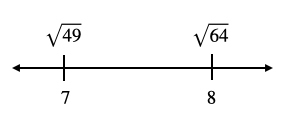407 493 6601

# 8th Grade - Irrational Numbers

## Introduction

• The real number system can be divided into two categories:
1. Rational Numbers
2. Irrational Numbers

## Irrational Numbers

• Real numbers that can't be expressed as a ratio of integers (such as $\frac{p}{q}$ where $p$ and $q$ are integers, $q\ne 0$) are called irrational numbers
• In simple terms, real numbers that are not rational are called irrational numbers.
• Some examples of irrational numbers are $\mathrm{\pi }$$\sqrt{2}$$e$ (Euler's number), etc.

## Properties of Irrational Numbers

• Irrational numbers are real numbers.
• The decimal expansion of an irrational number is always non-terminating & non-recurring.
• Example: the value of $\mathrm{\pi }=$3.141592......
• If $x$ is rational and $y$ is irrational, then $x+y$ and $x-y$ are irrational numbers. Also, $xy$ and $\frac{x}{y}$ are irrational numbers, $y\ne 0$.

## Approximating Values of Irrational Numbers

• Let us understand the process of evaluating the approximate value of an irrational number by the example below.

Question: Approximate the value of $\sqrt{50}$.

Solution:

• Identify the perfect squares less than and greater than $\sqrt{50}$, and locate them on a number line.$\sqrt{49}<\sqrt{50}<\sqrt{64}$ or $7<\sqrt{50}<8$

• $\sqrt{50}$ lies between the whole numbers 7 and 8. $\sqrt{50}$ is closer to 49. Hence, the whole number approximation of $\sqrt{50}$ is 7.
• To find the decimal component of the approximation, use the hit & trial method.

Try 7.1

${\left(7.1\right)}^{2}=50.41$$\approx 50$

• Another method of approximating the value of an irrational number is by using the shortcut formula $\sqrt{x}\approx \frac{x+y}{2\sqrt{y}}$. This is used to compute the approximate square root of a non-perfect square number. The number $y$ is the nearest perfect square number to the number $x$.
• To calculate the approximate cube root of a non-perfect cube number, we can use the shortcut formula $\sqrt{b}\approx \frac{2b+a}{2a+b}\sqrt{a}$ where $a$ is the nearest perfect cube number to the number $b$.

## Solved Examples

Question 1: Order the given numbers from least to greatest.

$0.25$$-\sqrt{10}$$-\frac{13}{2}$, and $\sqrt{50}$

Solution: The whole number approximation of $\sqrt{10}=3$, and $\sqrt{50}=7$. Also, $\frac{13}{2}=6.5$.

Let us now arrange the given numbers based on the information above.

$-\frac{13}{2}<-\sqrt{10}<0.25<\sqrt{50}$

Question 2: Fill in the blank using an appropriate symbol ($<$$>$$=$).

Solution: The whole number approximation of $\sqrt{26}=5$. Also, $2\mathrm{\pi }-1=\left(2×3.14\right)-1$$=6.28-1=5.28$

Thus,

## Cheat Sheet

• Irrational numbers are real numbers.
• Irrational numbers can't be expressed as a ratio of two integers.
• Irrational numbers are non-terminating and non-repeating (recurring) decimals.
• The square root of a non-perfect square is an irrational number.
• π (Pi) is a famous irrational number. The decimal approximation of π is 3.14.
• Use approximation to estimate the value of the square root of a non-perfect square.

## Blunder Areas

• The product of $\mathrm{\pi }×\mathrm{\pi }$ is ${\mathrm{\pi }}^{2}$, which is an irrational number.
• The product of $\sqrt{2}×\sqrt{2}$ is 2, which is a rational number.